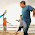World's most popular travel blog for travel bloggers.

# What is the utility of Bezier Curves in Computer Graphics? Write the properties of the Bezier curves and prove all properties. What do you understand by the Parametric Continuities and Geometric Continuities in context of Bezier Curves? Discuss both type of continuities with suitable expressions, equations and examples. Explain the role of control points in Bezier Curves. Draw a Bezier curve having the control points as P0(0, 0), P1(2, 5), P2(5, 9), P3(10, 20). Calculate the coordinates of the points on the curve corresponding to the parameter u = 0.2, 0.4, 0.6. Draw a rough sketch of the curve and show coordinates of various points on it?

, ,

## Bézier curve

A Bezier curve is a mathematically defined curve used in two-dimensional graphic applications. The curve is defined by four points: the initial position and the terminating position (which are called "anchors") and two separate middle points (which are called "handles"). The shape of a Bezier curve can be altered by moving the handles. The mathematical method for drawing curves was created by Pierre Bézier in the late 1960's for the manufacturing of automobiles at Renault.

Bezier curves have the following properties −
• They generally follow the shape of the control polygon, which consists of the segments joining the control points.
• They always pass through the first and last control points.
• They are contained in the convex hull of their defining control points.
• The degree of the polynomial defining the curve segment is one less that the number of defining polygon point. Therefore, for 4 control points, the degree of the polynomial is 3, i.e. cubic polynomial.
• A Bezier curve generally follows the shape of the defining polygon.
• The direction of the tangent vector at the end points is same as that of the vector determined by first and last segments.
• The convex hull property for a Bezier curve ensures that the polynomial smoothly follows the control points.
• No straight line intersects a Bezier curve more times than it intersects its control polygon.
• They are invariant under an affine transformation.
• Bezier curves exhibit global control means moving a control point alters the shape of the whole curve.
• A given Bezier curve can be subdivided at a point t = t0 into two Bezier segments which join together at the point corresponding to the parameter value t = t0.
• A very useful property of a Bezier curve is that it always passes through the first and last control points. That is, the boundary conditions at the two ends of the curve are P (0) =p0
P (1) =p_n
• Values of the parametric first derivatives of a Bezier curve at the endpoints can he calculated from control-point co-ordinates as
${P}^{\prime }\left(0\right)=-np0+np1$
${P}^{\prime }\left(1\right)=-np\left(n-1\right)+n{p}_{n}$
Thus, the slope at the beginning of the curve is along the line joining the first two control points, and the slope at the end of the curve is along the line joining the last two endpoints. Similarly, the parametric second derivatives of a Bezier curve at the endpoints are calculated as
${P}^{″}\left(0\right)=n\left(n-1\right)\left[\left(p2-p1\right)-\left(p1-p0\right)\right]$
${P}^{″}\left(1\right)=n\left(n-1\right)\left[p\left(n-2\right)-p\left(n-1\right)-\left(p\left(n-1\right)-{p}_{n}\right)\right]$
Another important property of any Bezier curve is that it lies within the convex hull (convex polygon boundary) of the control points. This follows from P3 the properties of Bezier blending functions: They are all positive and their sum is always 1,
${\sum }_{k=0}^{n}BEZ\left(u\right)=1$
So, that any curve position is simply the weighted sum of the control-point p s i - Positions. The context-hull property for a Bezier curve ensures that the polynomial smoothly follows the control points without erratic oscillations.

#### 1 comment:

1.can you share me link which contains all question present

Let us know your responses and feedback